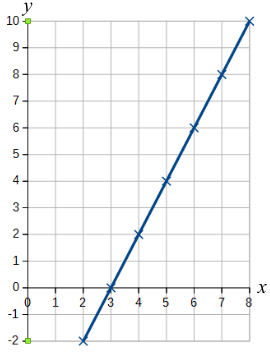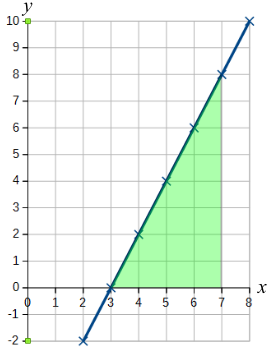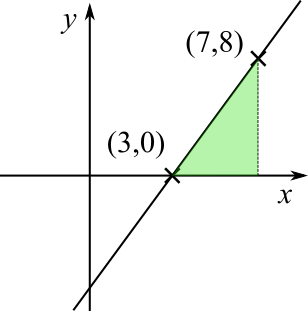#### You may also like### Consecutive Numbers

An investigation involving adding and subtracting sets of consecutive numbers. Lots to find out, lots to explore.### Calendar Capers

Choose any three by three square of dates on a calendar page...### Days and Dates

Investigate how you can work out what day of the week your birthday will be on next year, and the year after...

# Graph Area

##### Age 11 to 14 ShortChallenge Level

Plotting the graph using a table of values
If we plot the graph first, then we will be able to see the shape whose area we are looking for.

We can use a table of values to plot the graph, and we are interested in $x$ values between $3$ and $7$, so going from $2$ to $8$ will help us see the whole shape.

If $x=2$, then $y=2\times2-6=4-6=-2$. The rest of the values can be found in the same way.

 $x$ $2$ $3$ $4$ $5$ $6$ $7$ $8$ $y$ $-2$ $0$ $2$ $4$ $6$ $8$ $10$

The graph looks like this:We need to find the area between $x=3$ and $x=7$, which is coloured green below.The shape is a right-angled triangle, and its base is $4$ units and its height is $8$ units. So its area is $\frac{1}{2}\times4\times8=16$ square units.

Sketching the graph using relevant points
From the equation $y=2x-6$, we know that the graph will be a straight line.

Since we are interested in the area between $x=3$ and $x=7$, we could check where the graph is at those two points.

When $x=3$, $y=2\times3-6=6-6=0$.
When $x=7$, $y=2\times7-6=14-6=8$.

So $(3,0)$ and $(7,8)$ lie on the graph.So the graph must be the straight line through those points, and the area required is the green area, as shown below.The shape is a right-angled triangle, and its base is $4$ units and its height is $8$ units. So its area is $\frac{1}{2}\times4\times8=16$ square units.

You can find more short problems, arranged by curriculum topic, in our short problems collection.